Home | | Finite Element Analysis | Finite Element Formulation of Boundary Value Problems

# Finite Element Formulation of Boundary Value Problems

Introduction, 1 A Brief History Of The Fem, 2 General Methods Of The Finite Element Analysis, 3 General Steps Of The Finite Element Analysis, 4 Objectives Of This Fem, 5 Applications Of Fem In Engineering, Weighted Residual Method, The General Weighted Residual Statement, Weak Formulation Of The Weighted Residual, Piece Wise Continuous Trial Function, Examples Of A Bar Finite Element, 1 Rigid Body, Principle Of Stationery Total Potential Pstp), 1 Potential Energy In Elastic Bodies, 2 Principle Of Minimum Potential Energy, Rayleigh – Ritz Method (Variational Approach), Advantages Of Finite Element Method, Disadvantages Of Finite Element Method.

FINITE ELEMENT FORMULATION OF BOUNDARY VALUE PROBLEMS

INTRODUCTION

The finite element method constitutes a general tool for the numerical solution of partial differential equations in engineering and applied science.

The finite element method (FEM), or finite element analysis (FEA), is based on the idea of building a complicated object with simple blocks, or, dividing a complicated object into small and manageable pieces. Application of this simple idea can be found everywhere in everyday life as well as in engineering.

Examples:

Lego (kidsplay) Buildings

Approximation of the area of a circle:Why Finite Element Method?

·        Design analysis:  hand calculations, experiments, and computer simulations

·        FEM/FEA is the most widely applied computer simulation method in engineering

1.A Brief History of the FEM

·        1943 --- Courant (variational method)

·        1956 --- Turner, clough, martin and top(stiffness)

·        1960 --- Clough (finite element plan problems)

·        1970 --- Applications on mainframe computer

·        1980 --- Microcomputers, pre and post processors

·        1990 --- Analysis of large structural systems

2.General Methods of the Finite Element Analysis

2. Force Method Internal forces are considered as the unknowns of the problem.

3.                Displacement or stiffness method Displacements of the nodes are considered as the unknowns of the problem.

3.General Steps of the Finite Element Analysis

·          Discretization of structure

·            Numbering of Nodes and Elements

·          Selection of Displacement function or interpolation function

·          Define the material behavior by using Strain Displacement and Stress Strain relationships

·          Derivation of element stiffness matrix and equations

·          Assemble the element equations to obtain the global or total equations

·          Applying boundary conditions

·          Solution for the unknown displacements computation of the element strains and stresses from the nodal displacements

·          Interpret the results (post processing).

4.Objectives of This FEM

·        Understand the fundamental ideas of the FEM

·        Know the behavior and usage of each type of elements covered in this course

·        Be able to prepare a suitable FE model for given problems

·        Can interpret and evaluate the quality of the results (know the physics of the problems)

·        Be aware of the limitations of the FEM (dont misuse the

·        FEM - a numerical tool)

5.Applications of FEM in Engineering

·        Mechanical/Aerospace/Civil/Automobile Engineering Structure analysis (static/dynamic, linear/nonlinear) Thermal/fluid flows

·        Electromagnetics

·        Geomechanics

·        Biomechanics

WEIGHTED RESIDUAL METHOD

It is a powerful approximate procedure applicable to several problems. For non structural problems, the method of weighted residuals becomes very useful. It has many types. The popular four methods are,

1. Point collocation method,

Residuals are set to zero at n different locations Xi, and the weighting function wi is denoted as d(x - xi).roblem I

Find the solution for the following differential equation.Problem 2

The differential equation of a physical phenomenon is given byObtain one term approximate solution by using galerkin method Solution:

Here the boundary conditions are not homogeneous so we assume a trial function as, y=a1x(x-1)+x

first we have to verify whether the trial function satisfies the boundary condition or not y=a1x(x-1)+x

when x=0, y=0 x=1, y=1

Resuldual R:So one of the approximate solution is,

y= 0.83x(x-1)+x = 0.83x2-0.83x+x

y=0.83 x2+0.17x

Problem 3

Find the deflection at the center of a simply supported beam of span length l subjected to uniform distributed load throughout its length as shown using (a) point collection method (b) Sub-

domain method    (c)least squared and (d) galerkin’n method.

Solution:THE GENERAL WEIGHTED RESIDUAL STATEMENT

After understanding the basic techniques and successfully solved a few pro blem general weighted residual statement can b e written as

R dx=0 for i= 1,2,…..n

Where wi=Ni

The better result will be obtained by considering more terms in polynomial and trigonometric series.

WEAK FORMULATI ON OF THE WEIGHTED RESIDUAL STATEMENT.

The analysis in Section as applied to the model problem provides an attra ctive perspective to the solution of certain partial differential equations: the solution is identified w ith a point, which minimizes an appropriately constructed functional over an admis- sible function space. Weak (variational) forms can be made fully equivalent to respective strong forms , as evidenced in the discussion of the weighted resid ual methods, under certain smoothness assumptions. However, the equivalence between weak (variati onal) forms and variational principles is not gu aranteed: indeed, there exists no general method of construct-

ing functionals I [u], whos e extremization recovers a desired weak (variational) form. In this

sense, only certain partial d ifferential equations are amenable to analysis and solution by variational methods.

Vainbergs theorem provides the necessary and sufficient condition for the equivalence of a weak (variational) form to a functi onal extremization problem. If such equivalenc e holds, the functional is referred to as a potential.

Theorem (Vainberg)

Consider a weak (variational) form

G(u, δu) := B(u, δu) + (f, δu) + (q¯ , δu)Γq  = 0 ,

where u ∈ U , δu ∈ U0 , and f and q¯ are independent of u. Assume th at G pos- sesses a Gˆateaux derivative in a neighb orhood N of u, and the Gˆateaux differen- tial Dδu1 B(u, δu2) is

continuous  in u at every point  of N .

Then, the necessary and sufficient  condition for the above weak form to b e derivable from a

potential in N is that

Dδu1 G(u, δu2) = Dδu2 G(u, δu1) ,

Namely that Dδu1 G(u, δu2) be symmetric for all δu1, δu2 =  U0 and all u = N .

Preliminary to proving the above theorem, introduce the following two lemmas:

Lemma 1 Show that   Dv I[u]  =        lim

In the above derivation, no te that operations   and |ω=0 are not interchan geable (as they

both refer to the same variable ω), while lim∆ω→0 and |ω=0 are interchangeable, conditional upon sufficient smoothness of I [u].

Lemma 2 (Lagrange’s formula)

Let I [u] be a functional with Gateaux derivatives everywhere, and u, u + δu be any points of U. Then,

I [u + δu] − I [u ] = Dδu I [u + ǫ δu]      0 < ǫ < 1.

To prove Lemma 2, fix u and u + δu in U, and define function f on R as

f(ω)  :=  I[u +  ω δu] .

It follows thatWhere Lemma 1 was inv oked. Then, u s i n g the standard mean-value theorem of calculus,

PIECE WISE CONTINUOUS TRIAL FUNCTION

In weighted residual method the polynomial and trigonometric series are u sed as trial function. This trial function is a single composite function and it is valid over the entire solution domain this assumed trial function solution should match closely to the exact solution of the differential equation and the boundary conditions, it i s nothing but a process of curve fitting. This curve fitting is carried out by piecewise method i.e., the more numbers of piece leads better curve fit. P iecewise method can be explained by the following si mple problem.

We know that the straight line can be drawn through any two points. Let, ƒ(x)=sin is the approx imated function for straight line segments.One straight line segment

Two straight line segment

One Spring ElementTwo nodes: i, j

Nodal displacements:     ui,      uj (in, m, mm)

Nodal forces:        fi,      fj (lb, Newton) Spring constant (stiffness):   k  (lb/in,

N/m, N/mm)

Spring force-displacement relationship: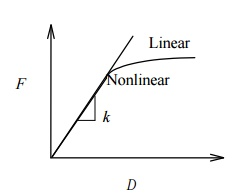k   F / (> 0) is the force needed to produce a unit stretch.

We only consider linear problems in this introductory course. Consider the equilibrium of forces for the spring.

At node 1 we haveor, where

(element) stiffness matrix

u = (element nodal) displacement vector

f  = (element nodal) force vector

Note:

That k is symmetric. Is k singular or non singular? That is, can we solve the equation? If not, why?

Problem 4

To find the deformation of the shape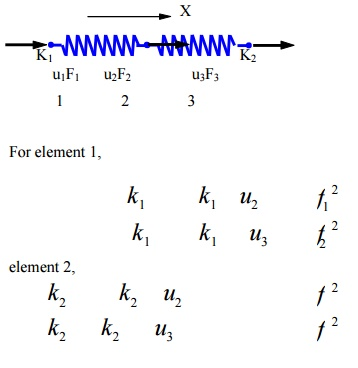where  fI at  node 2 F2

M is the (internal) force acting on local node i of element Consider the quilibrium of forces at node

Checking the Results

Deformed shape of the structure

Balance of the external forces

Order of magnitudes of the numbers

Suitable for stiffness analysis

Not suitable for stress analysis of the spring itself

Can have spring elements with stiffness in the lateral direction, Spring elements for torsion, etc.

EXAMPLES OF A BAR FINITE ELEMENT

The finite element method can be used to solve a variety of problem types in engineering, mathematics and science. The three main areas are mechanics of materials, heat transfer and fluid mechanics. The one-dimensional spring element belongs to the area of mechanics of materials, since it deals with the displacements, deformations and stresses involved in a solid body subjected to external loading.

Element dimensionality:

An element can be one-dimensional, two-dimensional or three-dimensio nal. A spring element is classified as one-dimensional.

Geometric shape of the element

The geometric shape of element can be represented as a line, area, or volume. The one-dimensional spring element is defi ned geometrically as: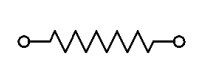Spring law

The spring is assumed to b e linear. Force (f) is directly proportional to deformation (Δ) via the spring constant k, i.e.Types of degrees of freedom per n ode

Degrees of freedom are displacements and/or rotations that are associated with a node. A one-dimensional spring element has two translational degrees of freedom, whi ch include, an axial (horizontal) displacement (u) at ea ch node.Element formulation

There are various ways to mathematically formulate an element. The simplest and limited approach is the direct method. More mathematically complex and general ap proaches are energy (variation) and weighted residual methods.

The direct method uses the fundamentals of equilibrium, compatibility and spring law from a sophomore level mechanics of material course. We will use the direct method to formulate the one-dimensional spring element because it is simple and based on a physical approach.

The direct method is an excellent setting for becoming familiar with such basis concepts of linear algebra, stiffness, degrees of freedom, etc., before using the mathematical formulation approaches as energy or weighted residuals.

Assumptions

Spring deformation

The spring law is a linear force-deformation as follows:

f = k

f - Spring Force (units: force)

k - Spring Constant (units: force/length)

- Spring Deformation (units: length)

Spring Behaviour:

A spring behaves the same in tension and compression.

Spring Stiffness:

Spring stiffness k is always positive, i.e., k>0, for a physical linear system.

Nodal Force Direction:

Loading is uniaxial, i.e., the resultant force is along the element. Spring has no resistance to lateral force.

Weightless Member:

Element has no mass (weightless).

Node Location:

The geometric location of nodes I and J cannot coincide, i.e., xi ≠ xj. The length of the element is only used to visually see the spring.

A column of KE is a vector of nodal loads that must be applied to an element to sustain a deformed state in which responding nodal DOF has unit value and all other nodal DOF are zero. In other words, a column of KE represents an equilibrium problem.

Example, uI = 1, uJ = 0.

Spring element has one rigid body mode.

Inter-Element Axial Displacement

The axial displacement (u ) is continuous through the assembled mesh a nd is described by a linear polynomial within each el ement. Each element in the mesh may be described by a different linear polynomial, depending on t he spring rate (k), external loading, and constraints on the element.

Inter-Element Deformation

The deformation (Δ) is pi ecewise constant through the assembled mesh and is described by a constant within each element. Ea ch element in the mesh may be described by a different constant, depending on the spring constant ( k), external loading, and constraints on the elem ent.

Inter-Element Internal Axial Forc e

The internal axial force (f) is piecewise continuous through the assembled mesh and is described by a constant within eac h element. Each element in the mesh may be described by a different constant, depending on the spring constant, external loading, and constraints on the element.1 Rigid Body

A body is considered rigi d if it does not deform when a force is applied. Consider rigid and non-rigid bars subjected to a graduually applied axial force of increasing magnitud e as shown.

The reader should note the followiing characteristics of rigid and non-rigid (flexib le) bodies:

·        Force Magnitude - Even if forces are large, a rigid body does not deform. A non-rigid body will deform even if a force is s mall. In reality, all bodies deform.

·        Failure - A rigid body does not fail under any load; while a non-rigid bod y will result either in

ductile or brittle failure w hen the applied load causes the normal stress t o exceed the breaking (fracture) stressb of the material. Brittle failure occurs when the applied load on the non-rigid bar shown above causes the breaking strength of the bar to be exceeded.

·        Material - The material is not considered in a rigid body. Since a rigid bod y does not deform (= 0) this is equivalent to an infinite modulus of elasticity. In contrast the modulus of elasticity

for a non-rigid material is finite, e.g., for steel, Esteel = 30 x 106 psi. (20 0 GPa). For rigid and non-rigid bars the material laws are:Rigid Body Motion

Rigid body motion occurs when forces and/or moments are applied to an unrestrained mesh (body), resulting in motion that occurs without any deformations in the entire m esh (body). Since no strains (deformations) occur during rigid body motion, there can be no stresses developed in the mesh.

A rigid body in general can be subjected to three types of motion, w hich are translation, rotation about a fixed axis, and general motion which consists of a combination o f both translation and rotation. These three motion types are as follows:

Translation - If any line segment on the body remains parallel to its original direction during the motion, it is said to be in translation. When the path of motion is along a straight line, the motion is called rectilinear translation, while a curved path is considered as a curvilinear translation. The curvilinear motion shown below is a combination of two translational motions, o ne horizontal motion and one vertical motion.Rotation About a Fixe d Axis - If all the particles of a rigid body mov e along circular paths, except the ones which lie on the axis of rotation, it is said to be in rotation ab out a fixed axis.General Motion - Any motion of a rigid body that consists of the combination of both translations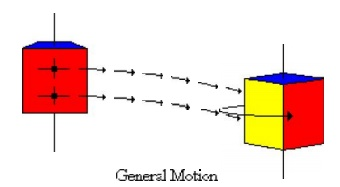There are six rigid bo dy modes in general three-dimensional situati on; three translational along the x, y, and z axes and three rotational about x, y, and z axes. Illustratio ns of these rigid body modes are presented as follows: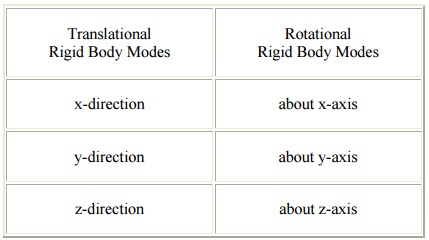Translational Rigid Body Modes :              Rotational Rigid Body Modes

Problem 6

A single 1-D 3-noded quadratic bar element has 3 nodes with local coordinates as shown in

FigureThe chosen approximation function for the field variable u is u = a + bx + cx2

Let the field variable u  have values u1 , u2 and u3 at nodes 1, 2 and 3, respectively.

To find the unknowns a, b and c, we apply the boundary conditionsSubstituting the values of a, b and c in equation (1) and collecting the coefficients of u1 , u 2  and u3

u = N1u1 + N 2 u 2 + N 3u3Derivation of stiffness matrix for 1-D 3-noded quadratic bar element:[D ] = E for a bar element (1-D case - only axial stress (s x ) and strain (e x ) exist Þ s x  = Ee x )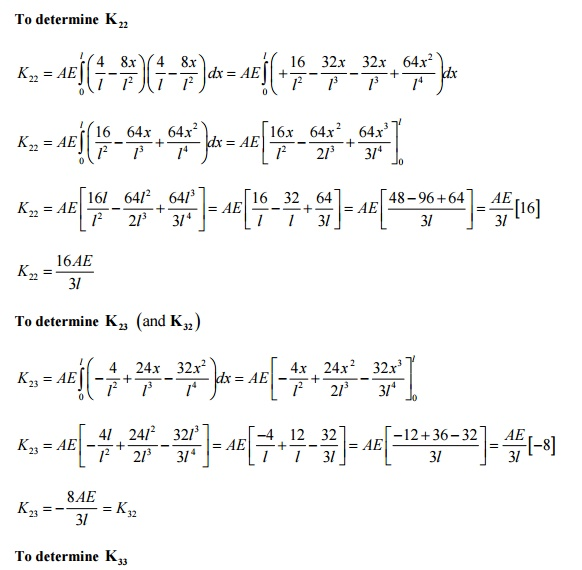PRINCIPLE OF STATIONERY TOTAL POTENTIAL (PSTP)

1 Potential energy in elastic bodies

Potential energy is the capacity to do the work by the force acting on deformable bodies; the forces acting on a body may be classified as external forces and internal forces. External forces are the applied loads while internal force is the stresses developed in the body. Hence the total potential energy is the sum of internal and external potential energy.

Consider a spring mass system let its stiffness be k and length L, due to a force P let it extend

by u

The load P moves down by distance u. hence it loses its capacity to do work by P u. the external potential energy in this case is given by.

H = -P u

Average force = Ku/2

The energy stored in the spring due to strain = Average force x Deflection

= Ku/2 x u

Total potential energy in the spring  =  K u2- P u

2 Principle of Minimum Potential Energy

From the expression for total potential energy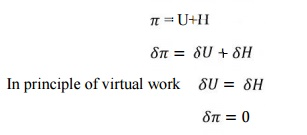Hence we can conclude that a deformable body is in equilibrium when the potential energy is having stationary value.

Hence the principle of minimum potential energy states among all the displacement equations that internal compatibility and the boundary condition those that also satisfy the equation of equilibrium make the potential energy a minimum is a stable system

Problem 7Given:         For the spring system shown above,

k1      100 N / mm,

k2      200 N / mm,

k3      100 N / mm

P       500 N,

u1      0

u4      0

Find: (a) The global stiffness matrix

(b)     Displacements of nodes 2 and 3

(c)      The reaction forces at nodes 1 and 4

(d)     the force in the spring 2

Solution:

(a)  The element stiffness matrices are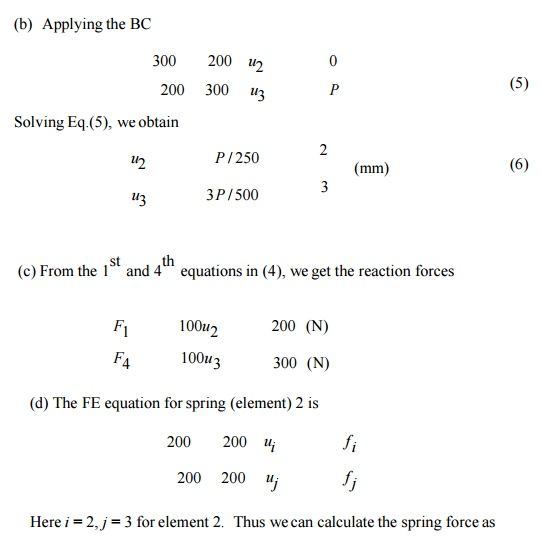Problem 8For the spring system with arbitrarily numbered nodes and elements, as shown above, find the global stiffness matrix.

Solution:

First we construct the following

Element Connectivity Table

Element      : Node i (1) : Node j (2)

1        4        2

2        2        3

3        3        5

4        2        1

Which specifies the global node numbers corresponding to the local node numbers for each element? Then we can write the element stiffness matrices as follows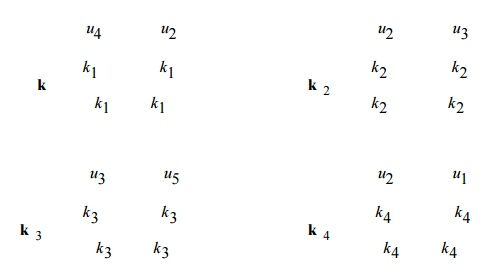Finally, applying the superposition method, we obtain the global stiffness matrix as follows

We may note that N1 and N2 obey the definition of shape function that is the shape function will have a value equal to unity at the node to which it belong and zero value at other  nodes.RAYLEIGH RITZ METHOD (VARIATIONAL APPROACH)

It is useful for solving complex structural problems. This method is possible only if a suitable functional is available. Otherwise, Galerkins method of weighted residual is used.

Problems (I set)

1. A simply supported beam subjected to uniformly distributed load over entire span. Determine the bending moment and deflection at midspan by using Rayleigh Ritz method and compare with exact solutions.

2. A bar of uniform cross section is clamed at one end and left free at another end and it is subjected to a uniform axial load P. Calculate the displacement and stress in a bar by using two terms polynomial and three terms polynomial. Compare with exact solutions.

1.    FEM can handle irregular geometry in a convenient manner.

2.    Handles general load conditions without difficulty

3.    Non homogeneous materials can be handled easily.

4.    Higher order elements may be implemented.

5.    It requires a digital computer and fairly extensive

6.    It requires longer execution time compared with FEM.

7.    Output result will vary considerably.

Study Material, Lecturing Notes, Assignment, Reference, Wiki description explanation, brief detail
Mechanical : Finite Element Analysis : Finite Element Formulation of Boundary Value Problems : Finite Element Formulation of Boundary Value Problems |